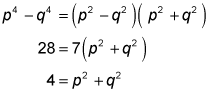##### SAT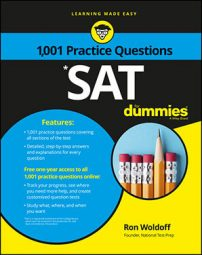The SAT Math exam contains questions where you'll need to know how to factor down a quadratic equation. The following practice questions ask you to do that, and then to plug in your solution to solve a given expression.

## Practice questions

1. What is the value of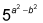if ab = 1/3 and a + b = 6?
2. What is the value of p2 + q2 if p2q2 = 7 and p4q4 = 28? A. 2 B. 3 C. 4 D. 5

1. The correct answer is 25. Remember that a2 b2 = (a + b)(ab). Plug in the values of a + b and ab to solve for a2b2: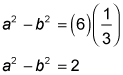Now plug 2 in for a2b2: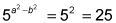2. The correct answer is Choice (C). Start by factoring p4q4: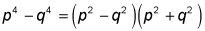Now plug in the values that the question gives you: